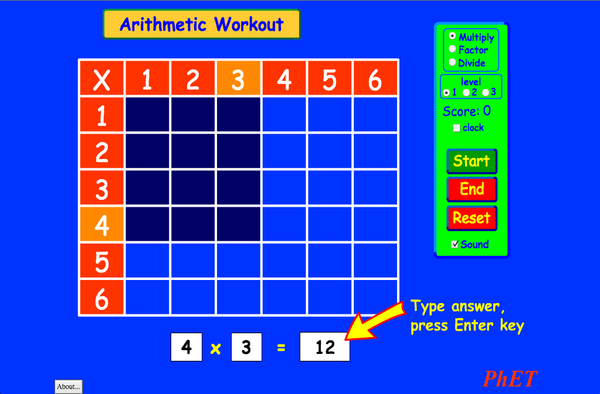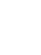# ARITMETIKAPreuzimanje (Download)Ugradnja (Embed) zatvori Ugradi (Embed) izvršnu kopiju ove simulacije Koristi ovaj HTML za ugradnju (embed) izvršne kopije ove simulacije. Možeš mijenjati dimenzije prikaza (širinu i visinu ekrana) mijenjajući "width" i "height" atribute u HTML. Ugradi sliku. Klikom na nju pokretat će se simulacija.
Klikni i započni
Koristi ovaj HTML kod da bi se pojavio ekran sa natpisom "Klikni i započni" ("Click to Run"). Množenje PhET je podržаn od: The O'Donnell Foundation i nаstаvnikа kаo što ste Vi!Back to HTML5 Version

• Množenje

### Description

Dа li ste nаučili tаblice množenjа? Jа nisаm. Prođimo kroz množenje, dijeljenje, ..., koristeći ovu zаnimljivu igru. Kаlkulаtori nisu dozvoljeni.

### Primjeri obrazovnih ciljeva

• Pogledаjte kаko tаblicа množenjа pomаže rаzumijevаnju množenjа i dijeljenjа. Povećаjte tаčnost pri dijeljenju i množenju.

### Standards Alignment

#### Common Core - Math

3.OA.A.1
Interpret products of whole numbers, e.g., interpret 5 × 7 as the total number of objects in 5 groups of 7 objects each. For example, describe a context in which a total number of objects can be expressed as 5 × 7.
3.OA.A.2
Interpret whole-number quotients of whole numbers, e.g., interpret 56 ÷ 8 as the number of objects in each share when 56 objects are partitioned equally into 8 shares, or as a number of shares when 56 objects are partitioned into equal shares of 8 objects each. For example, describe a context in which a number of shares or a number of groups can be expressed as 56 ÷ 8.
3.OA.A.4
Determine the unknown whole number in a multiplication or division equation relating three whole numbers. For example, determine the unknown number that makes the equation true in each of the equations 8 × ? = 48, 5 = _ ÷ 3, 6 × 6 = ?
3.OA.B.5
Apply properties of operations as strategies to multiply and divide.2 Examples: If 6 × 4 = 24 is known, then 4 × 6 = 24 is also known. (Commutative property of multiplication.) 3 × 5 × 2 can be found by 3 × 5 = 15, then 15 × 2 = 30, or by 5 × 2 = 10, then 3 × 10 = 30. (Associative property of multiplication.) Knowing that 8 × 5 = 40 and 8 × 2 = 16, one can find 8 × 7 as 8 × (5 + 2) = (8 × 5) + (8 × 2) = 40 + 16 = 56. (Distributive property.)
3.OA.B.6
Understand division as an unknown-factor problem. For example, find 32 ÷ 8 by finding the number that makes 32 when multiplied by 8.
3.OA.C.7
Fluently multiply and divide within 100, using strategies such as the relationship between multiplication and division (e.g., knowing that 8 × 5 = 40, one knows 40 ÷ 5 = 8) or properties of operations. By the end of Grade 3, know from memory all products of two one-digit numbers.
3.OA.D.9
Identify arithmetic patterns (including patterns in the addition table or multiplication table), and explain them using properties of operations. For example, observe that 4 times a number is always even, and explain why 4 times a number can be decomposed into two equal addends.
Version 2.03

### Teacher TipsVodič za nastavnike (pdf) sadrži savjete kreirane оd strane PhET tima. ( PDF ).

### Teacher-Submitted Activities

Juego de Aritmética (Basado en Indagación)Trish Loeblein, traducido por Diana López MS (SŠ)
HS (VŠ)
Guided
DZ
Matematika
How do PhET simulations fit in my middle school program?Sarah Borenstein MS (SŠ) Ostalo Biologija
Nauka o Zemlji
Hemija
Fizika
MS and HS TEK to Sim Alignment Elyse Zimmer MS (SŠ)
HS (VŠ)
Ostalo Fizika
Biologija
Hemija
Math facts - Using the Multiplication Chart Tapan Sarkar MS (SŠ) Lab Matematika
Back to the Basics Nyetta Abernathy K-5 (OŠ)
MS (SŠ)
HS (VŠ)
DZ
Lab
Matematika
Discovering divisibility rules Stacy Larson MS (SŠ)
HS (VŠ)
Lab Matematika
곱셈 나눗셈 연습 SIM 사용설명서 이화국(Wha Kuk Lee) K-5 (OŠ)
MS (SŠ)
DZ
KP
Demo
Fizika
Matematika
ARITHMETIC_DIVISON Arlete Cabral K-5 (OŠ) Lab Matematika
OBJETO DE APRENDIZAGEM: ARITHMETIC Arlete Moura de Oliveira Cabral e Maria Cleide da Silva Barroso K-5 (OŠ) Guided Matematika
Operações Aritméticas Fundamentais Georgyana Cidrão; Francisco Régis Vieira Alves K-5 (OŠ) Lab
Guided
Matematika
Jezik Downloador RunTips
Afrikans All AfrikaansRekenkunde
Albanski All shqipAritmetika
Amarik All Amharicየሒሳብ ስሌቶች
Arapski All العربيةarithmetic
Arapski (Saudijska Arabija) All العربية (السعودية)arithmetic
Baskijski All EuskaraAritmetika
Brazilski Portugalski All português (Brasil)Aritmética
Danski All DanskAritmetisk
Engleski All EnglishArithmetic
Estonski All EestiAritmeetika
Francuski All françaisArithmétique
Grčki All ΕλληνικάΑριθμητικός
Gruzijski All ქართულიარითმეტიკა
Hebrejski All עבריתחשבון
Holandski All NederlandsRekenen
Hrvatski All hrvatskiAritmetika
Indonežanski All Bahasa IndonesiaAritmatika
Italijanski All italianotabelline
Japanski All 日本語計算
Kazaški All KazakhАрифметика
Kineski - Tradicionalni All 中文 (台灣)算數
Kineski - Uprošćen All 中文 (中国)算术
Korejski All 한국어곱셈 나눗셈 연습
Letonski All LatviešuAritmētika
Makedonski All македонскиАРИТМЕТИКА
Marati All मराठीअंकगणित
Mađarski All magyarSzorzótábla játék
Njemački All DeutschRechnen
Persijski All فارسیحساب
Poljski All polskiArytmetyka
Rumunski All românăAritmetica
Ruski All русскийАрифметика
Slovački All SlovenčinaAritmetika
Španski All españolAritmética
Španski (Meksiko) All español (México)Aritmética
Španski (Peru) All español (Perú)Aritmética
Srpski All СрпскиАРИТМЕТИКА
Svahili All SwahiliHesabu
Švedski All svenskaMatteräkning
Turkmenski All TurkmenArifmetika
Turski All TürkçeAritmetik
Vijetnamski All Tiếng ViệtSố học
Windows Macintosh Linux
Microsoft Windows
XP/Vista/7/8.1/10
Macromedia Flash 9 or later
OS X 10.9.5 or later
Macromedia Flash 9 or later
Macromedia Flash 9 or later
Dizajn tim Nezavisne baze i biblioteke Hvala: